Display Accessibility Tools

# 6-4 Covering and Surrounding - Concepts and Explanations

## The Measurement Process

• Identify an object and the attribute you want to measure.
• Select an appropriate unit.
• Repeatedly "match" the unit to the attribute of the object.
• Determine the number of units

### Example

To find perimeter, count the number of linear units needed to surround an object.

To find area, count the number of square units needed to cover an object

## Area and Perimeter of Rectangles

To find the area, find the number of squares in one row (length) and multiply by the number of rows (width). So, the area formula is A = l x w.

To find the perimeter, add the length and width and double that sum. Or, calculate two lengths plus two widths. So, the perimeter formula is P = 2(l + w) or P = 2l + 2w

### Example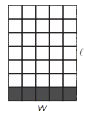There are 5 squares in the first row and 7 rows in all. The area of the rectangle is 5 x 7 = 35 square units.

The perimeter of the rectangle is 2(7 + 5) or 2 x 7 + 2 x 5 = 24 units.

## Area and Perimeter of Triangles

When you surround a triangle with a rectangle, you can see that the area of the triangle is half the area of the rectangle. You may turn the triangle to a convenient side as the base, if needed. So, the area formula is 1/2 b x h, where b is the base of the triangle (length of the rectangle) and h is the height of the triangle (width of the rectangle).

To find the perimeter, measure the lengths of the three sides and add them together.

### Example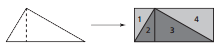Triangle 1 is congruent to Triangle 2. Triangle 3 is congruent to Triangle 4

.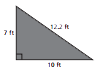The perimeter of the triangle is 7 + 10 + 12.2, or 29.2 ft

## Area and Perimeter of Parallelograms

When you draw a diagonal of a parallelogram, you form two congruent triangles. The parallelogram and triangle have the same base and height. So, the area formula is 2 x (1/2b x h) or b x h.

To find the perimeter, measure the lengths of the four sides and add them together.

### Example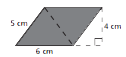The area of the parallelogram is 6 x 4 or 24 cm2.

The perimeter of the parallelogram is 2(5 + 6) or 2 x 5 + 2 x 6 = 22 cm.

## Volume of Right Rectangular Prism

Count the number of unit cubes needed to fill an object. Find the number of cubes in the base layer and multiply by the height. So, the volume formula is V = Bh, where B is the area of the base and h is the height. Or, you can multiply the three dimensions. So, another formula is V = l X w X h.

### Example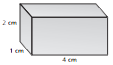The volume of the rectangular prism is 4(2) or 4 x 1 x 2 = 8 cm3.

## Surface Area of Prisms

Nets or “flat patterns” are two-dimensional representations of three-dimensional objects. To find the surface area of a prism, find the sum of the areas of each face of a net for that prism.

### Example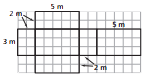S.A = (2 x 3) + (5 x 2) + (5 x 3) + (5 x 2) + (2 x 3) + (5 x 3) = 62m2

## Finding Lengths in the Coordinate Plane

If the x-coordinates (or y-coordinates) are the same for two points, you can find the distance between the points by finding the absolute value of the difference of their y-values (or x-values).

### Example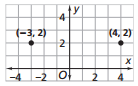The y-values are equal, so the distance between these points is | -3 -4 |, or 7 units.